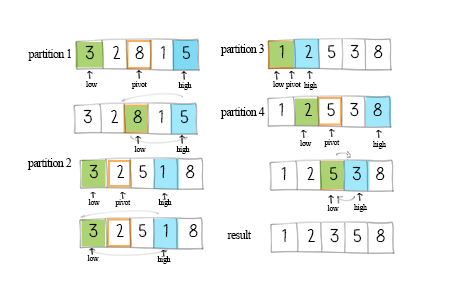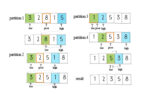Quicksort uses divide and conquer technique to provide fast sorting. Divide-and-conquer is a technique works by recursively breaking down a problem into two or more sub-problems of the same or related type, until these become simple enough to be solved directly.

### How quicksort works

First the sorting method calls a partition method to divide the array into two subarrays. This method returns an index for partition. Then the sorting method calls itself twice to sort these two subarrays.The key part of the solution is the partition method. The variable pivot determines when to swap unordered elements. Its initial value can be the first, last or the middle item in the subarray. In this solution, the pivot is the middle of the array. The formula of its index is mid=low+(high-low)/2.

There are two pointers, low points to the first element. high points to the last element. Increase the low pointer if the values it points are less than the pivot. Decrease the high pointer if the values are larger than the pivot. After moving the pointers, if the low pointer is still less equal than the high pointer, swap the elements in the array. After each partition, either the largest element swaps to the right, or the smallest element swaps to the left.

## Doodle